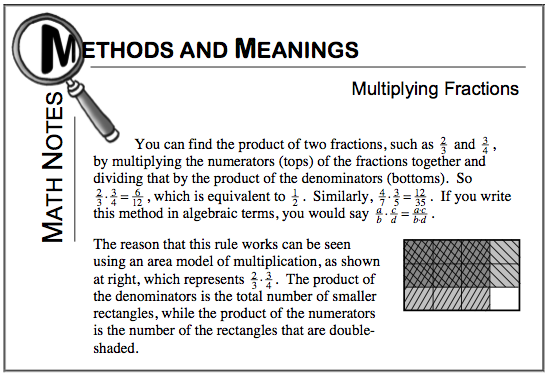### Home > CC1 > Chapter 6 > Lesson 6.1.3 > Problem6-36

6-36.

Multiply the following fractions. Homework Help ✎1. $\frac { 7 } { 8 } \cdot \frac { 5 } { 6 }$

Refer to the Math Notes box below if you are having trouble getting started.Multiply the numerators together and the denominators together.

$\left ( \frac{7}{8} \right )\left ( \frac{5}{6} \right )=\frac{(7)(5)}{(8)(6)}$

Now, simplify the fraction, if possible.

$\frac{35}{48}$

1. $\frac { 2 } { 13 } \cdot \frac { 4 } { 5 }$

Follow the steps from part (a) and refer to the Math Notes box if you are having trouble.

1. $\frac { 6 } { 7 } \cdot \frac { 6 } { 7 }$

$\frac{36}{49}$
1. $\frac { 4 } { 7 } \cdot \frac { 3 } { 8 }$